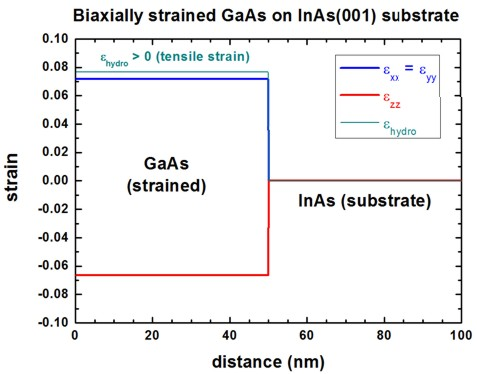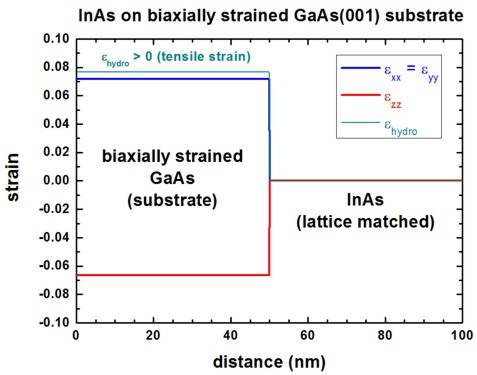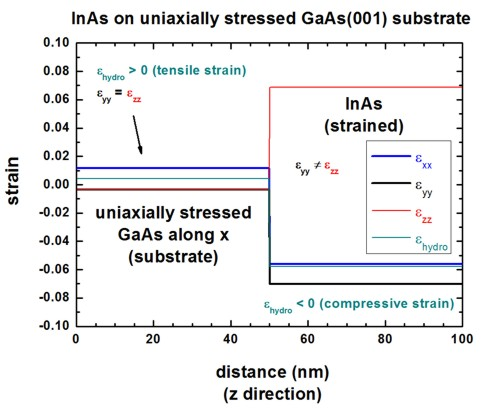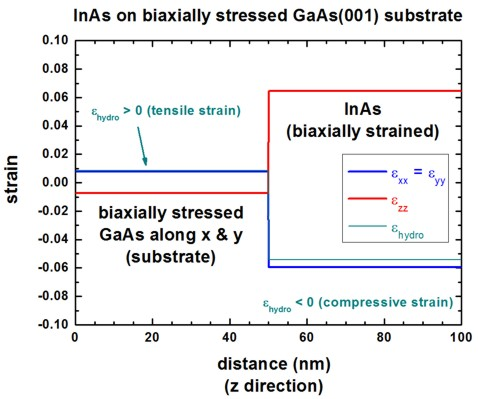nextnano.com  GUI: nextnanomat  Tool: nextnano++  Tool: nextnano³  Tool: nextnano.NEGF  Download | Search | Copyright | News | Publications  * password protected nextnano³ software1D strained substrate

# nextnano3 - Tutorial

## Growth of layers on strained (or stressed) substrates (biaxial and uniaxial)

Authors: Stefan Birner, Michael Povolotskyi

```-> 1D_GaAs_biaxial_on_InAs001.in -> 1D_InAs_on_biaxially_strainedGaAs001.in -> 1D_InAs_on_uniaxially_stressedGaAs001.in -> 1D_InAs_on_uniaxially_stressedGaAs001_input_stress.in -> 1D_InAs_on_biaxially_stressedGaAs001.in -> 1D_InAs_on_biaxially_stressedGaAs001_input_stress.in```

`==> ` Download these input files
`    `If you don't have a password yet, you have to first sign up for a free evaluation license in order to download these input files.

## Growth of layers on strained (or stressed) substrates (biaxial and uniaxial)

In this tutorial we show how to input a fixed strain tensor for a semiconductor starting layer in addition to its bulk lattice constants.
All other semiconductor layers that are grown on top are pseudomorphically strained with respect to the strained substrate layer.
In other words, the strain tensor of one specific layer is kept fixed whereas in all other layers the elastic energy is going to be minimized.

Material parameters of GaAs and InAs

Lattice constants
```GaAs: a = 0.565325 nm InAs: a = 0.60583  nm```

Elastic constants
```GaAs: c11 = 122.1  GPa      c12 = 56.6  GPa InAs: c11 =  83.29 GPa      c12 = 45.26 GPa```

## a) Biaxially strained GaAs on InAs(001) substrate

`-> 1D_GaAs_biaxial_on_InAs001.in`

If one assumes that GaAs is grown pseudomorphically with respect to an unstrained InAs(001) substrate along the z direction, then the following strain tensor components are obtained:

exx = eyy = e|| = ( asubstrate `-` alayer ) / alayer = 0.071649051

ezz = e_|_ = `-` D001 e|| = `-` 2 (c12/c11) e|| = `-` 0.066426474

ehydro = Tr(e) = exx + eyy + ezz = 0.076871628

Here, GaAs is biaxially strained and the overall strain is tensile (i.e. the hydrostatic strain ( Tr(eij) ) is positive) because GaAs has a smaller lattice constant than InAs.## b) InAs on biaxially strained GaAs(001) substrate

`-> 1D_InAs_on_biaxially_strainedGaAs001.in`

We now grow InAs pseudomorphically on a strained GaAs substrate where the GaAs substrate has the following strain tensor components:

``` \$strain-minimization-model  ...  input-substrate-strain      = yes             ! 'yes' = ```read in strain tensor components of substrate```  strain-epsilon-substrate-xx =  0.071649051d0  ! ```e||   of a)```  strain-epsilon-substrate-yy =  0.071649051d0  ! ```e||    of a)```  strain-epsilon-substrate-zz = -0.066426474d0  ! ```e_|_ of a)```  strain-epsilon-substrate-xy =  0d0            ! ```exy```  strain-epsilon-substrate-xz =  0d0            ! ```exz```  strain-epsilon-substrate-yz =  0d0            ! ```eyz``` ```The GaAs strain tensor components have been chosen in such a way, as if GaAs is biaxially strained with respect to an InAs(001) substrate, pseudomorphically grown along the z growth direction.

If one minimizes the elastic energy with respect to the strained GaAs substrate, then InAs is calculated to be unstrained, as one would expect.
Note that the GaAs substrate's lattice constants are kept fixed.

The same result would be obtained if` strain-epsilon-substrate-zz `takes an arbitrary value, as only the in-plane strain tensor components e|| are relevant.Obviously, the substrate can have any arbitrary strain tensor components, e.g. hydrostatic, biaxial, uniaxial or shear strain.

## c) InAs on uniaxially and biaxially strained GaAs(001) substrate

Again, the material system is an InAs layer grown on a GaAs(001) substrate that itself is subject to a variable uniaxial or biaxial stress.
We first apply a tensile uniaxial stress of 1 GPa ( = 10 kbar) on the GaAs substrate followed by a tensile biaxial stress of 1 GPa ( = 10 kbar).
Since nextnano³ accepts as inputs the strain tensor components of the substrate (and not the stress), we first have to calculate these components based on uniaxial and biaxial stresses of 1 GPa.

• Tensile, uniaxial stress of 1 GPa: (sigmaxx = 1 GPa, all other stress tensor components of sigma are zero.)

a) Reading in the strain tensor
``` -> 1D_InAs_on_uniaxially_stressedGaAs001.in ```To calculate the strain tensor analytically, we use the following equation:

sigmai = Eij . epsilonj         (i,j = 1...6)         (matrix-vector notation of Hooke's law, Voigt notation, Eij is the elasticity matrix)

In this particular example (uniaxial for zinblende) it holds for the three unknows exx, eyy, ezz:
(Note that all shear components are zero for this case.)

sigmax = sigmaxx = c11 * epsilon1 +  c12 * epsilon2 + c12 * epsilon3 =
= c11 * exx         +  c12 * eyy        + c12 * ezz = 1 GPa

sigmay = sigmayy = c12 * epsilon1 +  c11 * epsilon2 + c12 * epsilon3 =
= c12 * exx         +  c11 * eyy        + c12 * ezz = 0

sigmaz = sigmazz = c12 * epsilon1 +  c12 * epsilon2 + c11 * epsilon3 =
= c12 * exx         +  c12 * eyy        + c11 * ezz = 0

These are three equations with three unknowns whereas due to symmetry arguments, it additionally holds: eyy = ezz

`==> `exx = sigmaxx / [c11 ``` -``` 2  c122 / (c11 + c12) ] = 0.011594748
`==> `eyy = ezz = `-` c12 / (c11 + c12) * exx = `-`0.003672427

Thus the following strain tensor corresponds to a strained GaAs substrate with a uniaxial stress along the x direction of 1 GPa:

``` input-substrate-strain      = yes             ! 'yes' = ```read in strain tensor components of substrate```  strain-epsilon-substrate-xx =  0.011594748d0  ! ```exx``` strain-epsilon-substrate-yy = -0.003672427d0  ! ```eyy``` strain-epsilon-substrate-zz = -0.003672427d0  ! ```ezz``` strain-epsilon-substrate-xy =  0d0            ! ```exy``` strain-epsilon-substrate-xz =  0d0            ! ```exz``` strain-epsilon-substrate-yz =  0d0            ! ```eyz``` ```This corresponds to a tensile, hydrostatic strain in GaAs of ehydro = 0.00424989.``` ```Then the InAs will be strained with respect to a uniaxially stressed GaAs substrate.
Thus the InAs has the following strain tensor components:

exx = `-`0.0560392
eyy = `-`0.0702856
ezz = ` `0.0686452

This corresponds to a compressive, hydrostatic strain in InAs of ehydro = `-`0.0576795.``````b) Reading in the stress tensor``` -> 1D_InAs_on_uniaxially_stressedGaAs001_input_stress.in ```An alternative way to get the same result, is to specify the stress tensor rather than the strain tensor:```  input-substrate-stress    = yes    ! 'yes' = ```read in stress tensor components of substrate```  stress-sigma-substrate-xx = 1d9    ! [Pa]  ! ```If GaAs is uniaxially stressed with sigmaxx = 1 GPa along the x direction.` stress-sigma-substrate-yy = 0d0    ! ` sigmayy = 0``` stress-sigma-substrate-zz = 0d0    ! ```sigmazz = 0``` stress-sigma-substrate-xy = 0d0    ! ```sigmaxy = 0``` stress-sigma-substrate-xz = 0d0    ! ```sigmaxz = 0``` stress-sigma-substrate-yz = 0d0    ! ```sigmayz = 0```  ```
• Tensile, biaxial stress of 1 GPa: (sigmaxx = sigmayy = sigma|| = 1 GPa = 10 kbar, all other stress tensor components of sigma are zero.)

`-> 1D_InAs_on_biaxially_stressedGaAs001.in`

The formulas (similar as above) lead to the following analytical equations:

`==> `exx = eyy = e|| = sigma|| c11` /` (2  c122 `-` c112  `-` c11c12) = 0.007922321
`==> `ezz = e_|_ = `-` D001 e|| = `-` 2 (c12/c11) e|| = `-`0.007344854

Thus the following strain tensor corresponds to a strained GaAs substrate with a biaxial stress along the x and y directions of 1 GPa:

``` input-substrate-strain      = yes             ! 'yes' = ```read in strain tensor components of substrate```  strain-epsilon-substrate-xx =  0.007922321d0  ! ```exx = e||``` strain-epsilon-substrate-yy =  0.007922321d0  ! ```eyy = e||``` strain-epsilon-substrate-zz = -0.007344854d0  ! ```ezz = e_|_``` strain-epsilon-substrate-xy =  0d0            ! ```exy``` strain-epsilon-substrate-xz =  0d0            ! ```exz``` strain-epsilon-substrate-yz =  0d0            ! ```eyz``` ```This corresponds to a tensile, hydrostatic strain in GaAs of ehydro = 0.00849979.``` ```Then the InAs will be strained with respect to the biaxially stressed GaAs substrate.
Thus the InAs has the following strain tensor components:

exx = `-`0.0594660
eyy = `-`0.0594660
ezz = ` `0.0646280

This corresponds to a compressive, hydrostatic strain in InAs of ehydro = `-`0.0543041.b) Reading in the stress tensor
``` -> 1D_InAs_on_biaxially_stressedGaAs001_input_stress.in ```An alternative way to get the same result, is to specify the stress tensor rather than the strain tensor:```  input-substrate-stress    = yes    ! 'yes' = ```read in stress tensor components of substrate```                                     !         ```If GaAs is biaxially stressed with ```  stress-sigma-substrate-xx = 1d9    ! [Pa]    ```sigmaxx = 1 GPa along the x direction and with.``` stress-sigma-substrate-yy = 1d9    ! [Pa]    ```sigmayy = 1 GPa along the y direction (sigmaxx = sigmayy).``` stress-sigma-substrate-zz = 0d0    ! [Pa]    ``` sigmazz = 0``` stress-sigma-substrate-xy = 0d0    ! [Pa]    ```sigmaxy = 0``` stress-sigma-substrate-xz = 0d0    ! [Pa]    ```sigmaxz = 0``` stress-sigma-substrate-yz = 0d0    ! [Pa]    ```sigmayz = 0

The features presented in this tutorial can be used to model stresses that often occur in the case of thermal expansion mismatches for III-V films grown on sapphire or silicon substrates.
The selective growth on pre-patterned mesas where local stress/strain variations obviously play a crucial role in the resulting electronic/optical properties is another possible application.

• Please help us to improve our tutorial. Send comments to``` support [at] nextnano.com```.